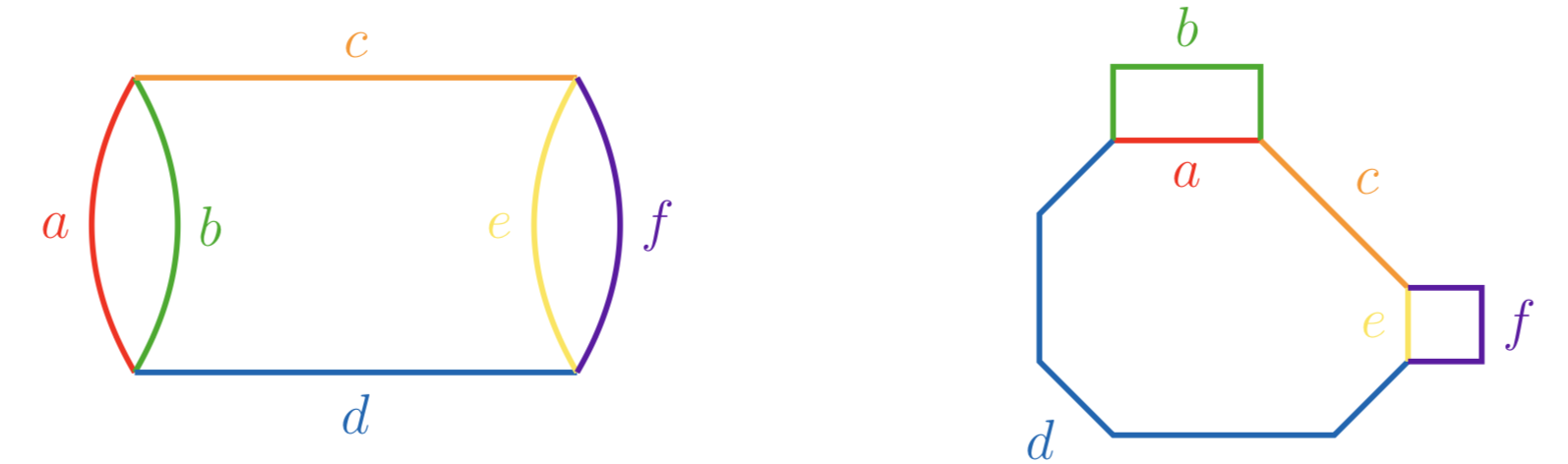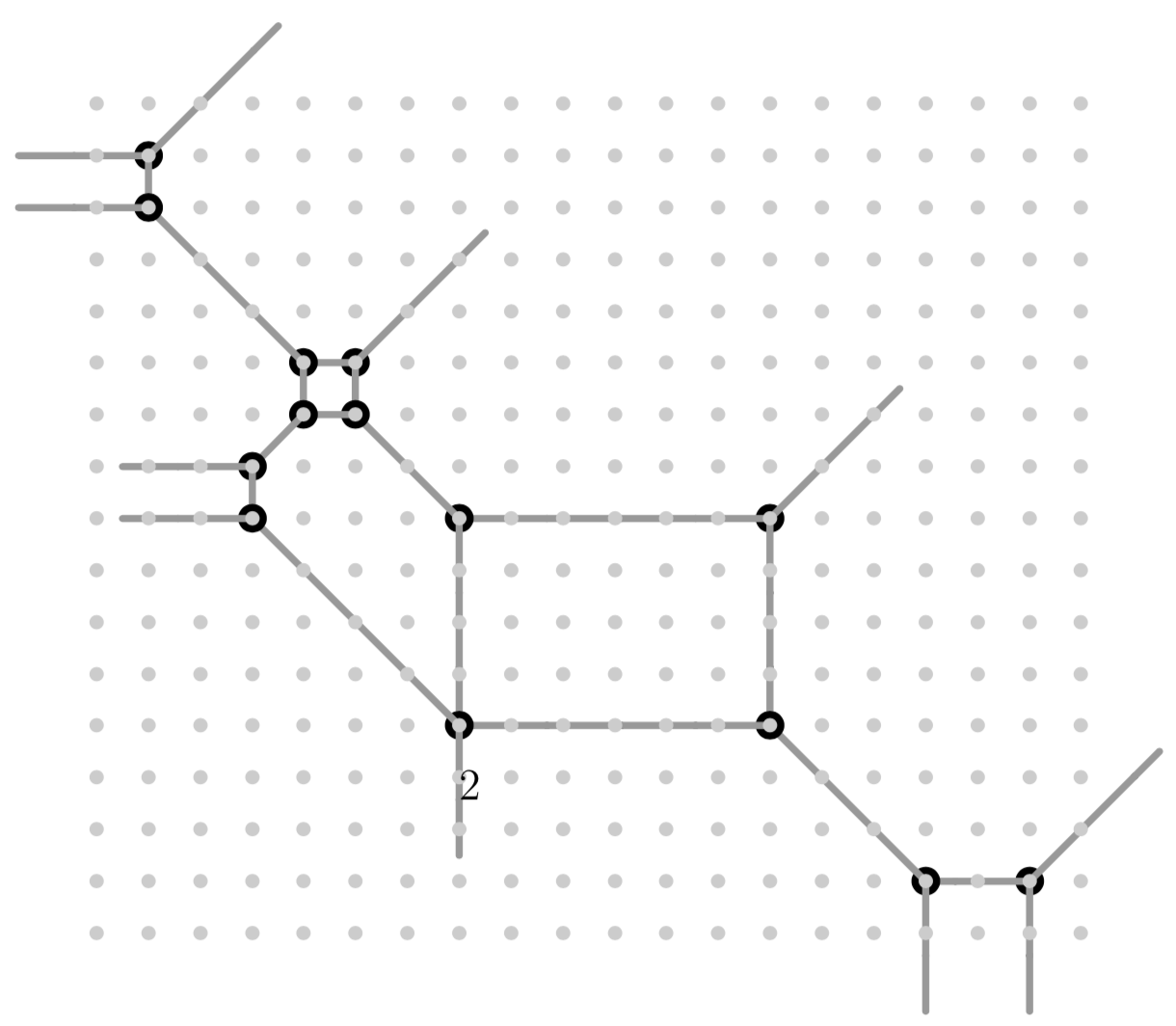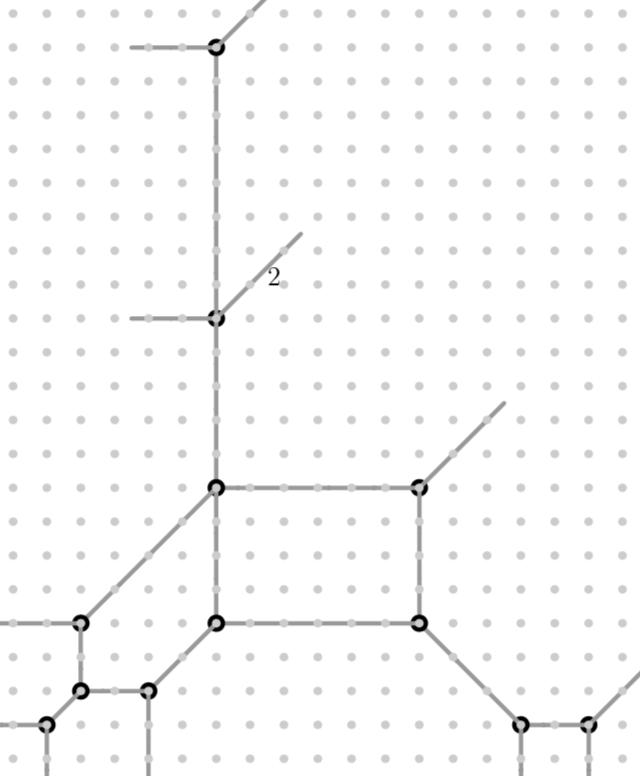# Type (020)¶

A tropical curve of type (020) is of the formDue to symmetry, we may assume that $$a\leq b$$, $$c\leq d$$ and $$e\leq f$$, as well as $$b-a\leq f-e$$. It can be realized as a plane tropical curve if and only if $$c + a \leq d$$ and $$c + e \leq d$$, and if one of two inequalities is an equality, and $$a=b$$, then $$a<e<f$$.

To illustrate how a curve is embeddable as a tropical quartic curve in a tropical plane, consider the following configuration of lengths which does not satisfy any of the previous three inequalities:

$a=1,\quad b=3,\quad c=2,\quad d=6,\quad e=10,\quad f=16,$

Consider the degenerate tropical curve given by the quartic polynomial

poly g = (1-2t9+3t20+t2+t7)+(2-3t9+4t20+t2)*x+x^2+(1-t6)*xy+x2y+t4x2y2+t6x3y+t9x3+t20x4+t4y+t9y2+t6xy2+t13xy3+t19y3+t30y4;
drawTropicalCurve(g,"max");

$\begin{split}g &= (1-2t^9+3t^{20}+t^2+t^7)+(2-3t^9+4t^{20}+t^2)\cdot x+x^2+(1-t^6)\cdot xy+x^2y\\ &\quad +t^4x^2y^2+t^6x^3y+t^9x^3+t^{20}x^4+t^4y+t^9y^2+t^6xy^2+t^{13}xy^3+t^{19}y^3+t^{30}y^4.\end{split}$It is has edge lengths

$a=1,\quad b=3,\quad c=2,\quad d=6,\quad e'=4,\quad f=16.$

and the modification $$z=x-1$$, which goes along $$w_x=0$$, yields the following tropical curve after projecting onto the $$zy$$ plane ($$y$$ remaining the vertical direction):

poly g1 = subst(g,x,x-1);
drawTropicalCurve(g1,"max");The entirety of $$w_x<0$$ is mapped onto $$w_z=0$$, while the edge on the line $$w_x=0$$ has been enlarged to length $$10$$.